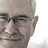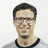### N:M relation in a view

There should be only one opening parenthesis between “unique” and “Œuvres”.Dear Alain, your diagram is perfect and, indeed, the problem was simply that of the parenthesis. I must admit that my base is far from being finished, at least with me as a developer.
I was wondering if you were a professional developer. If so, I would be happy to consider a business relationship with you to solve the remaining problems: establishing an annual inventory statement and finalizing the copyright calculation.
You can contact me by email: infoATnewconsonantmusic.com.
Thank you in any case for your past and present help.

I am not a professional developer, but a just retired ICT guy having fun with a new toy, and trying to give some help by sharing a few tricks... So, with this new year, I hope that I will be able to complement past and present help with some amount of future help.`alert(“Hello”)`I finally managed to fix the issue thanks to Alain (and the end of the year days-off). Here is my button script for anyboby needing some inspiration :

`let xCurrRec := ID;`
`let xYear := Year;`
`"// Select Medias ready for inventory //";`
`for i in select Medias where text(Step) = "Inventaire" and 'Année_sortie' <= xYear do`
`"// Create a subrecord for each Media //";`
`let x := (create InventaireLigne);`
`x.(Inventaire := xCurrRec);`
`x.(Year := xYear);`
`x.(Media := i);`
`"// *** variations annuelles *** //";`
`let xVentes := sum((select Ventes where Media = i and year(Factures.Date) = xYear).Nb);`
`x.(Nb_VentesAn := xVentes);`
`let yVentes := sum((select Ventes where Media = i and year(Factures.Date) = xYear).Prix_total);`
`x.(VentesAn := yVentes);`
`let xHommages := sum((select Hommages where Media = i and year(Date_hommage) = xYear).Nb);`
`x.(Nb_HommagesAn := xHommages);`
`let xTirages := sum((select Tirages where 'Médias' = i and Nb > 0 and year(Date_tirage) = xYear).Nb);`
`x.(Nb_TiragesAn := xTirages);`
`let xPilons := -1 * sum((select Tirages where 'Médias' = i and Nb < 0 and year(Date_tirage) = xYear).Nb);`
`x.(Nb_PilonsAn := xPilons);`
`let xFraisProduction := sum((select Tirages where 'Médias' = i and Nb > 0 and year(Date_tirage) = xYear).Cout);`
`x.(Frais_ProdAn := xFraisProduction);`
`"// *** état final du stock *** //";`
`let zVentes := sum((select Ventes where Media = i).Nb);`
`x.(Nb_VentesTot := zVentes);`
`let zHommages := sum((select Hommages where Media = i).Nb);`
`x.(Nb_HommagesTot := zHommages);`
`let zTirages := sum((select Tirages where 'Médias' = i and Nb > 0).Nb);`
`x.(Nb_TiragesTot := zTirages);`
`let zPilons := sum((select Tirages where 'Médias' = i and Nb < 0).Nb);`
`x.(Nb_PilonsTot := zPilons);`
`let zFraisProduction := sum((select Tirages where 'Médias' = i and Nb > 0).Cout);`
`x.(Frais_ProdTot := zFraisProduction)`
`end`

(Well, I have other small problems but this will be for a new thread...)A last (?) problem connected with this case...

I have to draw up a sales statement for each composer in order to calculate his yearly royalties. In the [Redevances] table, I have placed a button in charge of creating a sub-record [RedevancesLignes] for each [Media] of the composer (this works... thank you Alain!). The script will then search for the sales made for each media and calculate the number and the amount of sales [Ventes]. However, only certain sales are taken into account. I've been looking since yesterday why many sales are ignored. I see no difference between the selected sales and the ignored ones. Do you understand the error in my script?

[Ventes] is linked to [RedevancesLignes] which is a sub-table of [Redevances].

`let xCurrRec := ID;`
`let xYear := 'Année';`
`let zComposer := "-" + Composer_No + "-";`
`"// Select Medias in production for inventory //";`
`for i in (select Medias where text(Production_state) = "Inventaire")[contains(Compositeurs_No, zComposer)] do`
` "// Create a subrecord for each Redevance //";`
` let x := (create RedevancesLignes);`
` x.(Redevances := xCurrRec);`
` x.(Year := xYear);`
` let yComposer := Compositeurs.Nom;`
` x.(Composer := yComposer);`
` let yTitle := i.Titre;`
` x.(Title := yTitle);`
` let yPrice := i.'Prix_détail';`
` x.(Price := yPrice);`
` let yProrata := i.Prorata;`
` x.(Prorata := yProrata);`
` "// *** Calculate each royalties PROBLEM HERE *** //";`
` let xVentes := sum((select Ventes where Media = i and Hors_redevances = false and year(Factures.Date) = xYear).Nb);`
` x.(Sales := xVentes);`
` let xSalesValue := sum((select Ventes where Media = i and Hors_redevances = false and year(Factures.Date) = xYear).Prix_total);`
` let xRoyalties := xSalesValue / (10 * yProrata);`
` x.(Royalties := xRoyalties)`
`end`

It's hard to debug something without actually seeing it... I suppose that the schema has not changed, except that the "Sales" table is now called "Ventes", and the "Invoice" table is now called "Factures". What I would do to debug the issue would be to create a view and populate it with the problematic select statement. This would give a real-time view of the selected records. Then, since there are three conditions that must be met for a record to be selected, I would try to remove one of them to see what happens. Of course, too many records would then be selected, but by studying the actual selection one would be able to determine if the now removed condition is the culprit. Hope this helps.Indeed, I removed each condition (even rearranged them) without obvious solution (to my eyes). I put a copy of the base here if you have any time to investigate :

Not every composer has sales... so the best is to go to Compositeurs > Lysight > tag Redevances > 2020 > button [Créer redevances annuelles du compositeur] (2 minutes needed)

For example, the sales for "35 Rhythmic Studies" do not appear !

Still investigating...

Thank you for your time and concern!

The problem seems to come from the "Hors_redevances" field. This field is empty in some records of the "Ventes" table. You can correct the isue by updating those records with the value "no", and avoid future trouble by defining a default value - I suppose "no" - in the field definition.Indeed, there was the problem! The field looks the same with a negative value and no value at all. It's fantastic to have found this. Thank you very much!
Send me your postal address to info@newconsonantmusic.com. I'd be happy to send you some records... so that you see what you contributed to.
Thank you very much again.

1 2